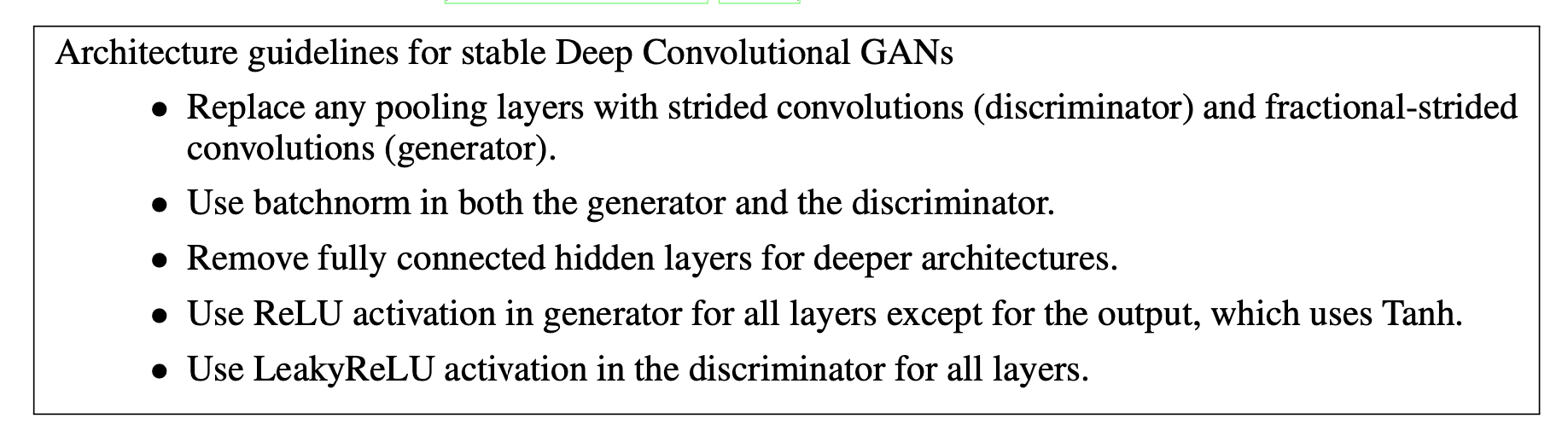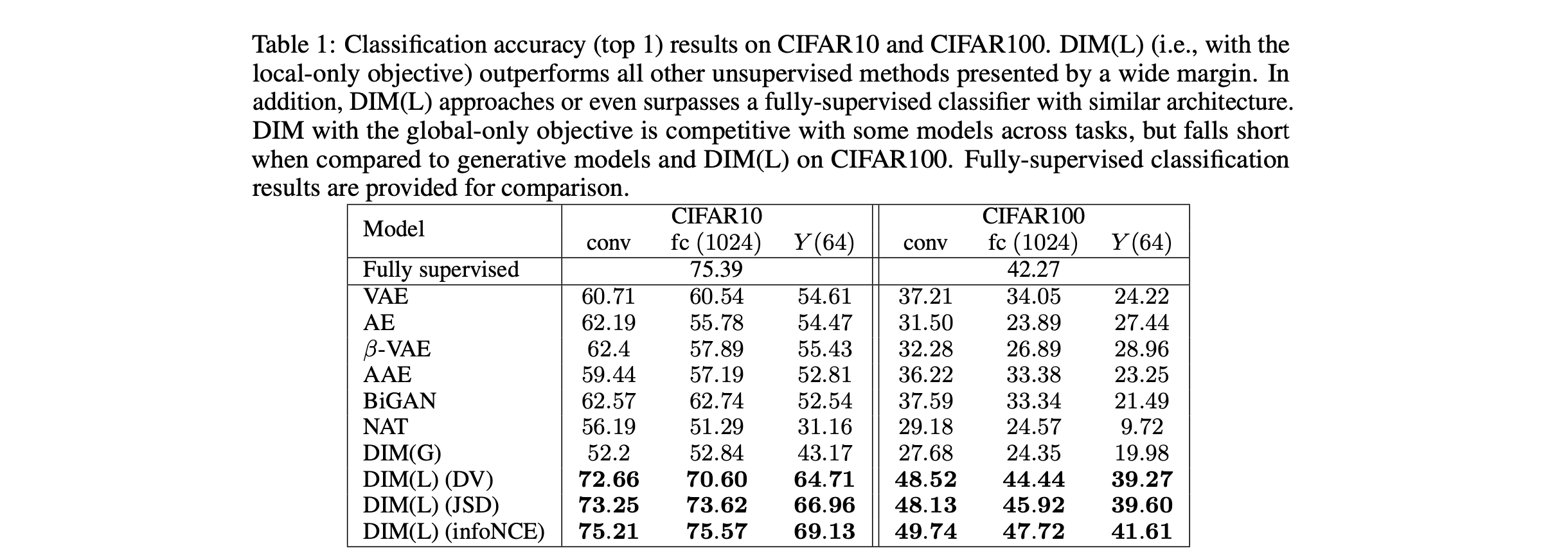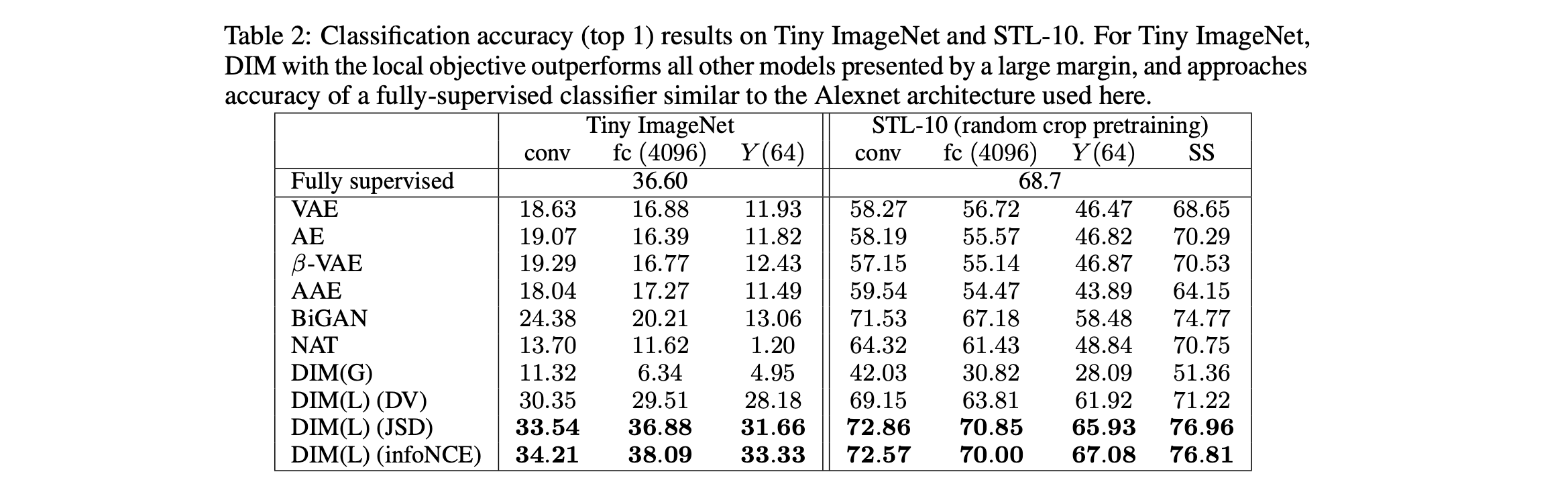N1H111SM's Miniverse

# Deep InfoMax

2020/05/21 ShareMaterials

• ICLR-2019 paper “LEARNING DEEP REPRESENTATIONS BY MUTUAL INFORMATION ESTIMATION AND MAXIMIZATION”
• ICLR-2016 paper “DCGAN”
• Pytorch DCGAN tutorial

# Motivation

Maximizing global MI is not sufficient to learn useful representations. We show that structure matters: incorporating knowledge about locality in the input into the objective can significantly improve a representation’s suitability for downstream tasks. We further control characteristics of the repre- sentation by matching to a prior distribution adversarially.

## Structure of Introduction

• We show that pure global MI maximization doesn’t learn useful representation.
• Structure matters: use global/local MI maximization improves the representation quality hugely.
• Representational characteristics like INDEPENDENCE is also very important. We combine MI maximization with prior matching in a manner similar to adversarial autoencoders (AAEs) to constrain representations according to desired statistical properties.

# Model Theory

## Global/local MI Maximization

The objective above can be used to maximize MI between input and output, but ultimately this may be undesirable depending on the tasks. Suppose the intermediate representation of the input preserves the characteristics of locality, which is represented as $C_{\psi}(x):=\left\{C_{\psi}^{(i)}\right\}_{i=1}^{M}$ , and $E_{\psi}(x)=f_{\psi} \circ C_{\psi}(x)$. Define our MI estimator on global/local pairs, maximizing the average estimated MI:

## Matching representations to a prior distribution

Absolute magnitude of information is only one desirable property of a representation; depending on the application, good representations can be compact, independent, disentangled, or independently controllable. 这一段话表明了其实关于representation quality本身的evaluation是多样的，对于不同的评估维度算法可能会有不同的表现。和VAE类似的是，文章将学到的representation的分布和自己预设的prior分布相匹配起来，通过类似于GAN的对抗生成训练方法来最小化两者之间的divergence.

## Final Optimization Objective

• Global-only objective DIM(G): $(\alpha, \beta, \gamma) = (1, 0, 1)$
• Local-only objective DIM(L): $(\alpha, \beta, \gamma) = (0, 1, 0.1)$

# Experiments

## Evaluation Methods

Linear classification. Using a support vector machine (SVM). This is simultaneously a proxy for MI of the representation with linear separability. 这是检验representation的linear separability的标准。

Non-linear classification. Using a single hidden layer neural network (200 units) with dropout. This is a proxy on MI of the representation with the labels separate from linear separability as measured with the SVM above.

Semi-supervised learning (STL-10 here). Fine-tuning the complete encoder by adding a small neural network on top of the last convolutional layer (matching architectures with a standard fully-supervised classifier). 通过unsupervised data来预训练数据的表示，然后将这部分网络连接到supervised training data上通过fine-tune达到更好的效果。

MS-SSIM (Wang et al., 2003). Using a decoder trained on the L2 reconstruction loss. This is a proxy for the total MI between the input and the representation and can indicate the amount of encoded pixel-level information.

Mutual information neural estimate (MINE). Class labels have limited use in evaluating representations, as we are often interested in information encoded in the representation that is unknown to us. However, we can use mutual information neural estimation to more directly measure the MI between the input and output of the encoder. 直接来estimate input $X$ 和 representation之间的MI.

Neural dependency measure (NDM). Using a second discriminator that measures the KL between representation and a batch-wise shuffled version of representation.

## Model Architecture### Discriminator Architecture

InfoMax需要将image往下压缩成representation，因此只需要关心discriminator的架构。

## Performance